# 3.1. Cross-validation: evaluating estimator performance¶

Learning the parameters of a prediction function and testing it on the same data is a methodological mistake: a model that would just repeat the labels of the samples that it has just seen would have a perfect score but would fail to predict anything useful on yet-unseen data. This situation is called overfitting. To avoid it, it is common practice when performing a (supervised) machine learning experiment to hold out part of the available data as a test set `X_test, y_test`. Note that the word “experiment” is not intended to denote academic use only, because even in commercial settings machine learning usually starts out experimentally.

In scikit-learn a random split into training and test sets can be quickly computed with the `train_test_split` helper function. Let’s load the iris data set to fit a linear support vector machine on it:

```>>> import numpy as np
>>> from sklearn.model_selection import train_test_split
>>> from sklearn import datasets
>>> from sklearn import svm

>>> iris.data.shape, iris.target.shape
((150, 4), (150,))
```

We can now quickly sample a training set while holding out 40% of the data for testing (evaluating) our classifier:

```>>> X_train, X_test, y_train, y_test = train_test_split(
...     iris.data, iris.target, test_size=0.4, random_state=0)

>>> X_train.shape, y_train.shape
((90, 4), (90,))
>>> X_test.shape, y_test.shape
((60, 4), (60,))

>>> clf = svm.SVC(kernel='linear', C=1).fit(X_train, y_train)
>>> clf.score(X_test, y_test)
0.96...
```

When evaluating different settings (“hyperparameters”) for estimators, such as the `C` setting that must be manually set for an SVM, there is still a risk of overfitting on the test set because the parameters can be tweaked until the estimator performs optimally. This way, knowledge about the test set can “leak” into the model and evaluation metrics no longer report on generalization performance. To solve this problem, yet another part of the dataset can be held out as a so-called “validation set”: training proceeds on the training set, after which evaluation is done on the validation set, and when the experiment seems to be successful, final evaluation can be done on the test set.

However, by partitioning the available data into three sets, we drastically reduce the number of samples which can be used for learning the model, and the results can depend on a particular random choice for the pair of (train, validation) sets.

A solution to this problem is a procedure called cross-validation (CV for short). A test set should still be held out for final evaluation, but the validation set is no longer needed when doing CV. In the basic approach, called k-fold CV, the training set is split into k smaller sets (other approaches are described below, but generally follow the same principles). The following procedure is followed for each of the k “folds”:

• A model is trained using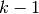of the folds as training data;
• the resulting model is validated on the remaining part of the data (i.e., it is used as a test set to compute a performance measure such as accuracy).

The performance measure reported by k-fold cross-validation is then the average of the values computed in the loop. This approach can be computationally expensive, but does not waste too much data (as it is the case when fixing an arbitrary test set), which is a major advantage in problem such as inverse inference where the number of samples is very small.

## 3.1.1. Computing cross-validated metrics¶

The simplest way to use cross-validation is to call the `cross_val_score` helper function on the estimator and the dataset.

The following example demonstrates how to estimate the accuracy of a linear kernel support vector machine on the iris dataset by splitting the data, fitting a model and computing the score 5 consecutive times (with different splits each time):

```>>> from sklearn.model_selection import cross_val_score
>>> clf = svm.SVC(kernel='linear', C=1)
>>> scores = cross_val_score(clf, iris.data, iris.target, cv=5)
>>> scores
array([ 0.96...,  1.  ...,  0.96...,  0.96...,  1.        ])
```

The mean score and the 95% confidence interval of the score estimate are hence given by:

```>>> print("Accuracy: %0.2f (+/- %0.2f)" % (scores.mean(), scores.std() * 2))
Accuracy: 0.98 (+/- 0.03)
```

By default, the score computed at each CV iteration is the `score` method of the estimator. It is possible to change this by using the scoring parameter:

```>>> from sklearn import metrics
>>> scores = cross_val_score(
...     clf, iris.data, iris.target, cv=5, scoring='f1_macro')
>>> scores
array([ 0.96...,  1.  ...,  0.96...,  0.96...,  1.        ])
```

See The scoring parameter: defining model evaluation rules for details. In the case of the Iris dataset, the samples are balanced across target classes hence the accuracy and the F1-score are almost equal.

When the `cv` argument is an integer, `cross_val_score` uses the `KFold` or `StratifiedKFold` strategies by default, the latter being used if the estimator derives from `ClassifierMixin`.

It is also possible to use other cross validation strategies by passing a cross validation iterator instead, for instance:

```>>> from sklearn.model_selection import ShuffleSplit
>>> n_samples = iris.data.shape
>>> cv = ShuffleSplit(n_splits=3, test_size=0.3, random_state=0)
>>> cross_val_score(clf, iris.data, iris.target, cv=cv)
...
array([ 0.97...,  0.97...,  1.        ])
```

Data transformation with held out data

Just as it is important to test a predictor on data held-out from training, preprocessing (such as standardization, feature selection, etc.) and similar data transformations similarly should be learnt from a training set and applied to held-out data for prediction:

```>>> from sklearn import preprocessing
>>> X_train, X_test, y_train, y_test = train_test_split(
...     iris.data, iris.target, test_size=0.4, random_state=0)
>>> scaler = preprocessing.StandardScaler().fit(X_train)
>>> X_train_transformed = scaler.transform(X_train)
>>> clf = svm.SVC(C=1).fit(X_train_transformed, y_train)
>>> X_test_transformed = scaler.transform(X_test)
>>> clf.score(X_test_transformed, y_test)
0.9333...
```

A `Pipeline` makes it easier to compose estimators, providing this behavior under cross-validation:

```>>> from sklearn.pipeline import make_pipeline
>>> clf = make_pipeline(preprocessing.StandardScaler(), svm.SVC(C=1))
>>> cross_val_score(clf, iris.data, iris.target, cv=cv)
...
array([ 0.97...,  0.93...,  0.95...])
```

### 3.1.1.1. The cross_validate function and multiple metric evaluation¶

The `cross_validate` function differs from `cross_val_score` in two ways -

• It allows specifying multiple metrics for evaluation.
• It returns a dict containing training scores, fit-times and score-times in addition to the test score.

For single metric evaluation, where the scoring parameter is a string, callable or None, the keys will be - `['test_score', 'fit_time', 'score_time']`

And for multiple metric evaluation, the return value is a dict with the following keys - `['test_<scorer1_name>', 'test_<scorer2_name>', 'test_<scorer...>', 'fit_time', 'score_time']`

`return_train_score` is set to `True` by default. It adds train score keys for all the scorers. If train scores are not needed, this should be set to `False` explicitly.

The multiple metrics can be specified either as a list, tuple or set of predefined scorer names:

```>>> from sklearn.model_selection import cross_validate
>>> from sklearn.metrics import recall_score
>>> scoring = ['precision_macro', 'recall_macro']
>>> clf = svm.SVC(kernel='linear', C=1, random_state=0)
>>> scores = cross_validate(clf, iris.data, iris.target, scoring=scoring,
...                         cv=5, return_train_score=False)
>>> sorted(scores.keys())
['fit_time', 'score_time', 'test_precision_macro', 'test_recall_macro']
>>> scores['test_recall_macro']
array([ 0.96...,  1.  ...,  0.96...,  0.96...,  1.        ])
```

Or as a dict mapping scorer name to a predefined or custom scoring function:

```>>> from sklearn.metrics.scorer import make_scorer
>>> scoring = {'prec_macro': 'precision_macro',
...            'rec_micro': make_scorer(recall_score, average='macro')}
>>> scores = cross_validate(clf, iris.data, iris.target, scoring=scoring,
...                         cv=5, return_train_score=True)
>>> sorted(scores.keys())
['fit_time', 'score_time', 'test_prec_macro', 'test_rec_micro',
'train_prec_macro', 'train_rec_micro']
>>> scores['train_rec_micro']
array([ 0.97...,  0.97...,  0.99...,  0.98...,  0.98...])
```

Here is an example of `cross_validate` using a single metric:

```>>> scores = cross_validate(clf, iris.data, iris.target,
...                         scoring='precision_macro')
>>> sorted(scores.keys())
['fit_time', 'score_time', 'test_score', 'train_score']
```

### 3.1.1.2. Obtaining predictions by cross-validation¶

The function `cross_val_predict` has a similar interface to `cross_val_score`, but returns, for each element in the input, the prediction that was obtained for that element when it was in the test set. Only cross-validation strategies that assign all elements to a test set exactly once can be used (otherwise, an exception is raised).

These prediction can then be used to evaluate the classifier:

```>>> from sklearn.model_selection import cross_val_predict
>>> predicted = cross_val_predict(clf, iris.data, iris.target, cv=10)
>>> metrics.accuracy_score(iris.target, predicted)
0.973...
```

Note that the result of this computation may be slightly different from those obtained using `cross_val_score` as the elements are grouped in different ways.

The available cross validation iterators are introduced in the following section.

## 3.1.2. Cross validation iterators¶

The following sections list utilities to generate indices that can be used to generate dataset splits according to different cross validation strategies.

### 3.1.2.1. Cross-validation iterators for i.i.d. data¶

Assuming that some data is Independent and Identically Distributed (i.i.d.) is making the assumption that all samples stem from the same generative process and that the generative process is assumed to have no memory of past generated samples.

The following cross-validators can be used in such cases.

NOTE

While i.i.d. data is a common assumption in machine learning theory, it rarely holds in practice. If one knows that the samples have been generated using a time-dependent process, it’s safer to use a time-series aware cross-validation scheme Similarly if we know that the generative process has a group structure (samples from collected from different subjects, experiments, measurement devices) it safer to use group-wise cross-validation.

#### 3.1.2.1.1. K-fold¶

`KFold` divides all the samples in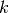groups of samples, called folds (if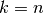, this is equivalent to the Leave One Out strategy), of equal sizes (if possible). The prediction function is learned usingfolds, and the fold left out is used for test.

Example of 2-fold cross-validation on a dataset with 4 samples:

```>>> import numpy as np
>>> from sklearn.model_selection import KFold

>>> X = ["a", "b", "c", "d"]
>>> kf = KFold(n_splits=2)
>>> for train, test in kf.split(X):
...     print("%s %s" % (train, test))
[2 3] [0 1]
[0 1] [2 3]
```

Each fold is constituted by two arrays: the first one is related to the training set, and the second one to the test set. Thus, one can create the training/test sets using numpy indexing:

```>>> X = np.array([[0., 0.], [1., 1.], [-1., -1.], [2., 2.]])
>>> y = np.array([0, 1, 0, 1])
>>> X_train, X_test, y_train, y_test = X[train], X[test], y[train], y[test]
```

#### 3.1.2.1.2. Repeated K-Fold¶

`RepeatedKFold` repeats K-Fold n times. It can be used when one requires to run `KFold` n times, producing different splits in each repetition.

Example of 2-fold K-Fold repeated 2 times:

```>>> import numpy as np
>>> from sklearn.model_selection import RepeatedKFold
>>> X = np.array([[1, 2], [3, 4], [1, 2], [3, 4]])
>>> random_state = 12883823
>>> rkf = RepeatedKFold(n_splits=2, n_repeats=2, random_state=random_state)
>>> for train, test in rkf.split(X):
...     print("%s %s" % (train, test))
...
[2 3] [0 1]
[0 1] [2 3]
[0 2] [1 3]
[1 3] [0 2]
```

Similarly, `RepeatedStratifiedKFold` repeats Stratified K-Fold n times with different randomization in each repetition.

#### 3.1.2.1.3. Leave One Out (LOO)¶

`LeaveOneOut` (or LOO) is a simple cross-validation. Each learning set is created by taking all the samples except one, the test set being the sample left out. Thus, for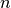samples, we havedifferent training sets anddifferent tests set. This cross-validation procedure does not waste much data as only one sample is removed from the training set:

```>>> from sklearn.model_selection import LeaveOneOut

>>> X = [1, 2, 3, 4]
>>> loo = LeaveOneOut()
>>> for train, test in loo.split(X):
...     print("%s %s" % (train, test))
[1 2 3] 
[0 2 3] 
[0 1 3] 
[0 1 2] 
```

Potential users of LOO for model selection should weigh a few known caveats. When compared with-fold cross validation, one buildsmodels fromsamples instead ofmodels, where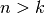. Moreover, each is trained on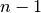samples rather than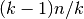. In both ways, assumingis not too large and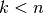, LOO is more computationally expensive than-fold cross validation.

In terms of accuracy, LOO often results in high variance as an estimator for the test error. Intuitively, sinceof thesamples are used to build each model, models constructed from folds are virtually identical to each other and to the model built from the entire training set.

However, if the learning curve is steep for the training size in question, then 5- or 10- fold cross validation can overestimate the generalization error.

As a general rule, most authors, and empirical evidence, suggest that 5- or 10- fold cross validation should be preferred to LOO.

References:

#### 3.1.2.1.4. Leave P Out (LPO)¶

`LeavePOut` is very similar to `LeaveOneOut` as it creates all the possible training/test sets by removing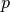samples from the complete set. Forsamples, this produces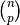train-test pairs. Unlike `LeaveOneOut` and `KFold`, the test sets will overlap for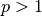.

Example of Leave-2-Out on a dataset with 4 samples:

```>>> from sklearn.model_selection import LeavePOut

>>> X = np.ones(4)
>>> lpo = LeavePOut(p=2)
>>> for train, test in lpo.split(X):
...     print("%s %s" % (train, test))
[2 3] [0 1]
[1 3] [0 2]
[1 2] [0 3]
[0 3] [1 2]
[0 2] [1 3]
[0 1] [2 3]
```

#### 3.1.2.1.5. Random permutations cross-validation a.k.a. Shuffle & Split¶

`ShuffleSplit`

The `ShuffleSplit` iterator will generate a user defined number of independent train / test dataset splits. Samples are first shuffled and then split into a pair of train and test sets.

It is possible to control the randomness for reproducibility of the results by explicitly seeding the `random_state` pseudo random number generator.

Here is a usage example:

```>>> from sklearn.model_selection import ShuffleSplit
>>> X = np.arange(5)
>>> ss = ShuffleSplit(n_splits=3, test_size=0.25,
...     random_state=0)
>>> for train_index, test_index in ss.split(X):
...     print("%s %s" % (train_index, test_index))
...
[1 3 4] [2 0]
[1 4 3] [0 2]
[4 0 2] [1 3]
```

`ShuffleSplit` is thus a good alternative to `KFold` cross validation that allows a finer control on the number of iterations and the proportion of samples on each side of the train / test split.

### 3.1.2.2. Cross-validation iterators with stratification based on class labels.¶

Some classification problems can exhibit a large imbalance in the distribution of the target classes: for instance there could be several times more negative samples than positive samples. In such cases it is recommended to use stratified sampling as implemented in `StratifiedKFold` and `StratifiedShuffleSplit` to ensure that relative class frequencies is approximately preserved in each train and validation fold.

#### 3.1.2.2.1. Stratified k-fold¶

`StratifiedKFold` is a variation of k-fold which returns stratified folds: each set contains approximately the same percentage of samples of each target class as the complete set.

Example of stratified 3-fold cross-validation on a dataset with 10 samples from two slightly unbalanced classes:

```>>> from sklearn.model_selection import StratifiedKFold

>>> X = np.ones(10)
>>> y = [0, 0, 0, 0, 1, 1, 1, 1, 1, 1]
>>> skf = StratifiedKFold(n_splits=3)
>>> for train, test in skf.split(X, y):
...     print("%s %s" % (train, test))
[2 3 6 7 8 9] [0 1 4 5]
[0 1 3 4 5 8 9] [2 6 7]
[0 1 2 4 5 6 7] [3 8 9]
```

`RepeatedStratifiedKFold` can be used to repeat Stratified K-Fold n times with different randomization in each repetition.

#### 3.1.2.2.2. Stratified Shuffle Split¶

`StratifiedShuffleSplit` is a variation of ShuffleSplit, which returns stratified splits, i.e which creates splits by preserving the same percentage for each target class as in the complete set.

### 3.1.2.3. Cross-validation iterators for grouped data.¶

The i.i.d. assumption is broken if the underlying generative process yield groups of dependent samples.

Such a grouping of data is domain specific. An example would be when there is medical data collected from multiple patients, with multiple samples taken from each patient. And such data is likely to be dependent on the individual group. In our example, the patient id for each sample will be its group identifier.

In this case we would like to know if a model trained on a particular set of groups generalizes well to the unseen groups. To measure this, we need to ensure that all the samples in the validation fold come from groups that are not represented at all in the paired training fold.

The following cross-validation splitters can be used to do that. The grouping identifier for the samples is specified via the `groups` parameter.

#### 3.1.2.3.1. Group k-fold¶

`GroupKFold` is a variation of k-fold which ensures that the same group is not represented in both testing and training sets. For example if the data is obtained from different subjects with several samples per-subject and if the model is flexible enough to learn from highly person specific features it could fail to generalize to new subjects. `GroupKFold` makes it possible to detect this kind of overfitting situations.

Imagine you have three subjects, each with an associated number from 1 to 3:

```>>> from sklearn.model_selection import GroupKFold

>>> X = [0.1, 0.2, 2.2, 2.4, 2.3, 4.55, 5.8, 8.8, 9, 10]
>>> y = ["a", "b", "b", "b", "c", "c", "c", "d", "d", "d"]
>>> groups = [1, 1, 1, 2, 2, 2, 3, 3, 3, 3]

>>> gkf = GroupKFold(n_splits=3)
>>> for train, test in gkf.split(X, y, groups=groups):
...     print("%s %s" % (train, test))
[0 1 2 3 4 5] [6 7 8 9]
[0 1 2 6 7 8 9] [3 4 5]
[3 4 5 6 7 8 9] [0 1 2]
```

Each subject is in a different testing fold, and the same subject is never in both testing and training. Notice that the folds do not have exactly the same size due to the imbalance in the data.

#### 3.1.2.3.2. Leave One Group Out¶

`LeaveOneGroupOut` is a cross-validation scheme which holds out the samples according to a third-party provided array of integer groups. This group information can be used to encode arbitrary domain specific pre-defined cross-validation folds.

Each training set is thus constituted by all the samples except the ones related to a specific group.

For example, in the cases of multiple experiments, `LeaveOneGroupOut` can be used to create a cross-validation based on the different experiments: we create a training set using the samples of all the experiments except one:

```>>> from sklearn.model_selection import LeaveOneGroupOut

>>> X = [1, 5, 10, 50, 60, 70, 80]
>>> y = [0, 1, 1, 2, 2, 2, 2]
>>> groups = [1, 1, 2, 2, 3, 3, 3]
>>> logo = LeaveOneGroupOut()
>>> for train, test in logo.split(X, y, groups=groups):
...     print("%s %s" % (train, test))
[2 3 4 5 6] [0 1]
[0 1 4 5 6] [2 3]
[0 1 2 3] [4 5 6]
```

Another common application is to use time information: for instance the groups could be the year of collection of the samples and thus allow for cross-validation against time-based splits.

#### 3.1.2.3.3. Leave P Groups Out¶

`LeavePGroupsOut` is similar as `LeaveOneGroupOut`, but removes samples related to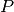groups for each training/test set.

Example of Leave-2-Group Out:

```>>> from sklearn.model_selection import LeavePGroupsOut

>>> X = np.arange(6)
>>> y = [1, 1, 1, 2, 2, 2]
>>> groups = [1, 1, 2, 2, 3, 3]
>>> lpgo = LeavePGroupsOut(n_groups=2)
>>> for train, test in lpgo.split(X, y, groups=groups):
...     print("%s %s" % (train, test))
[4 5] [0 1 2 3]
[2 3] [0 1 4 5]
[0 1] [2 3 4 5]
```

#### 3.1.2.3.4. Group Shuffle Split¶

The `GroupShuffleSplit` iterator behaves as a combination of `ShuffleSplit` and `LeavePGroupsOut`, and generates a sequence of randomized partitions in which a subset of groups are held out for each split.

Here is a usage example:

```>>> from sklearn.model_selection import GroupShuffleSplit

>>> X = [0.1, 0.2, 2.2, 2.4, 2.3, 4.55, 5.8, 0.001]
>>> y = ["a", "b", "b", "b", "c", "c", "c", "a"]
>>> groups = [1, 1, 2, 2, 3, 3, 4, 4]
>>> gss = GroupShuffleSplit(n_splits=4, test_size=0.5, random_state=0)
>>> for train, test in gss.split(X, y, groups=groups):
...     print("%s %s" % (train, test))
...
[0 1 2 3] [4 5 6 7]
[2 3 6 7] [0 1 4 5]
[2 3 4 5] [0 1 6 7]
[4 5 6 7] [0 1 2 3]
```

This class is useful when the behavior of `LeavePGroupsOut` is desired, but the number of groups is large enough that generating all possible partitions withgroups withheld would be prohibitively expensive. In such a scenario, `GroupShuffleSplit` provides a random sample (with replacement) of the train / test splits generated by `LeavePGroupsOut`.

### 3.1.2.4. Predefined Fold-Splits / Validation-Sets¶

For some datasets, a pre-defined split of the data into training- and validation fold or into several cross-validation folds already exists. Using `PredefinedSplit` it is possible to use these folds e.g. when searching for hyperparameters.

For example, when using a validation set, set the `test_fold` to 0 for all samples that are part of the validation set, and to -1 for all other samples.

### 3.1.2.5. Cross validation of time series data¶

Time series data is characterised by the correlation between observations that are near in time (autocorrelation). However, classical cross-validation techniques such as `KFold` and `ShuffleSplit` assume the samples are independent and identically distributed, and would result in unreasonable correlation between training and testing instances (yielding poor estimates of generalisation error) on time series data. Therefore, it is very important to evaluate our model for time series data on the “future” observations least like those that are used to train the model. To achieve this, one solution is provided by `TimeSeriesSplit`.

#### 3.1.2.5.1. Time Series Split¶

`TimeSeriesSplit` is a variation of k-fold which returns firstfolds as train set and the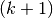th fold as test set. Note that unlike standard cross-validation methods, successive training sets are supersets of those that come before them. Also, it adds all surplus data to the first training partition, which is always used to train the model.

This class can be used to cross-validate time series data samples that are observed at fixed time intervals.

Example of 3-split time series cross-validation on a dataset with 6 samples:

```>>> from sklearn.model_selection import TimeSeriesSplit

>>> X = np.array([[1, 2], [3, 4], [1, 2], [3, 4], [1, 2], [3, 4]])
>>> y = np.array([1, 2, 3, 4, 5, 6])
>>> tscv = TimeSeriesSplit(n_splits=3)
>>> print(tscv)
TimeSeriesSplit(max_train_size=None, n_splits=3)
>>> for train, test in tscv.split(X):
...     print("%s %s" % (train, test))
[0 1 2] 
[0 1 2 3] 
[0 1 2 3 4] 
```

## 3.1.3. A note on shuffling¶

If the data ordering is not arbitrary (e.g. samples with the same class label are contiguous), shuffling it first may be essential to get a meaningful cross- validation result. However, the opposite may be true if the samples are not independently and identically distributed. For example, if samples correspond to news articles, and are ordered by their time of publication, then shuffling the data will likely lead to a model that is overfit and an inflated validation score: it will be tested on samples that are artificially similar (close in time) to training samples.

Some cross validation iterators, such as `KFold`, have an inbuilt option to shuffle the data indices before splitting them. Note that:

• This consumes less memory than shuffling the data directly.
• By default no shuffling occurs, including for the (stratified) K fold cross- validation performed by specifying `cv=some_integer` to `cross_val_score`, grid search, etc. Keep in mind that `train_test_split` still returns a random split.
• The `random_state` parameter defaults to `None`, meaning that the shuffling will be different every time `KFold(..., shuffle=True)` is iterated. However, `GridSearchCV` will use the same shuffling for each set of parameters validated by a single call to its `fit` method.
• To get identical results for each split, set `random_state` to an integer.

## 3.1.4. Cross validation and model selection¶

Cross validation iterators can also be used to directly perform model selection using Grid Search for the optimal hyperparameters of the model. This is the topic of the next section: Tuning the hyper-parameters of an estimator.